# Exercise E12.2 Heron’s Formula NCERT Solutions Class 9

## Chapter 12 Ex.12.2 Question 1

A park, in the shape of a quadrilateral $$ABCD,$$ has $$\angle C=90^\circ,$$   $$AB = 9 \;\rm{m}$$, $$BC = 12\;\rm{ m}$$, $$CD = 5 \;\rm{m}$$ and $$AD = 8\;\rm{m}$$. How much area does it occupy?

### Solution

What is known?

Dimensions of the quadrilateral

$$AB = 9 \;\rm{m}$$, $$BC = 12\;\rm{ m}$$, $$CD = 5 \;\rm{m}$$

and $$AD = 8 \;\rm{m}$$.

What is unknown?

Area of the park (quadrilateral).

Reasoning:

We can calculate the area of quadrilateral by dividing the quadrilateral into triangular parts and then use the Heron’s formula for area of the triangle.

The formula given by Heron about the area of a triangle

$$=\sqrt{s(s-a)(s-b)(s-c)}$$

Where $$a, b$$ and $$c$$ are the sides of the triangle, and

\begin{align}s &= \text{Semi-perimeter}\\& = \begin{Bmatrix} \text{Half the Perimeter } \\ \text{ of the triangle} \;\end{Bmatrix} \\&=\frac{(a+b+c)}{2}\end{align}

Steps:

$$ABCD$$ is park shown in the figure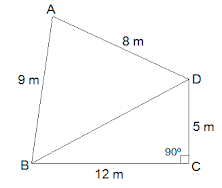We have,

$$\angle C$$ = $$90^\circ$$, $$AB = 9 \;\rm{m}$$, $$BC = 12\;\rm{ m}$$, $$CD = 5 \;\rm{m}$$

and $$AD = 8\; \rm{m}$$.

$$BD$$ is joined

In $$\Delta BDC$$, we have

\begin{align}&{BD^{2}=BC^{2}+CD^{2} \begin{bmatrix} \text { Pythagoras}\\ \text{ theorem } \end{bmatrix} } \\ &{BD^{2}=12^{2}+5^{2}} \\ &{BD^{2}=144+25} \\ &{BD=\sqrt{169}} \\ &{BD=13 \, \mathrm{m}}\end{align}

Area of  $$\Delta BDC$$

\begin{align}&=\frac{1}{2} \times \text { base } \times \text { height } \\ &=\frac{1}{2} \times 12 \times 5 \\ &=30 \mathrm{m}^{2} \end{align}

In $$\Delta ABD$$,

$$AB = a = \;9\;\rm{ m}$$, $$AD = b = \;8\;\rm{ m}$$, $$BD = c = \;13\;\rm{ m}$$

Semi Perimeter

\begin{align}s& =\frac{(a+b+c)}{2}\\ &=\frac{9+8+13}{2} \\ & =\frac{30}{2} \\&=15\, \mathrm{m}\end{align}

By using Heron’s formula,

Area of $$\Delta ABD$$

\begin{align}&={\sqrt{s(s-a)(s-b)(s-c)}} \\ &=\sqrt{ 15\! \times\! (6\!-\!9 ) (15\!-\!8) (15\!-\!13) } \\ &=\sqrt{15 \times 6 \times 7 \times 2} \\ &=\sqrt{1260}\;\mathrm{m}^{2} \end{align}

Area of a $$\Delta ABD$$ $$= 35.5 \;\rm{m^2}$$

Therefore area of park $$ABCD$$

$$=$$ Area of  $$\Delta \, BDC$$+ Area of  $$\Delta \, ABD$$

$$=30 \mathrm{m}^{2}+35.5 \mathrm{m}^{2}$$

Area of the park $$= 65.5 \;\rm{m^2}$$

## Chapter 12 Ex.12.2 Question 2

Find the area of a quadrilateral $$ABCD$$ in which $$AB = 3\;\rm{ cm}$$,$$BC= 4\;\rm{ cm}$$, $$CD= 4\;\rm{ cm}$$, $$DA = 5\;\rm{ cm}$$ and $$AC = 5\;\rm{cm}$$.

### Solution

What is known?

Dimensions of the quadrilateral

$$AB = 3\;\rm{ cm}$$$$BC= 4\;\rm{ cm}$$, $$CD= 4\;\rm{ cm}$$, $$DA = 5\;\rm{ cm}$$

and $$AC = 5\;\rm{cm}$$.

What is unknown?

Area of the quadrilateral.

Reasoning:

We can calculate the area of quadrilateral by dividing the quadrilateral into triangular parts and then use the Heron’s formula for area of the triangle.

The formula given by Heron about the area of a triangle

$$=\sqrt{s(s-a)(s-b)(s-c)}$$

Where $$a, b$$ and $$c$$ are the sides of the triangle, and

\begin{align}s &= \text{Semi-perimeter}\\& = \begin{Bmatrix} \text{Half the Perimeter } \\ \text{ of the triangle} \;\end{Bmatrix} \\&=\frac{(a+b+c)}{2}\end{align}

Steps:

$$ABCD$$ is quadrilateral shown in the figure.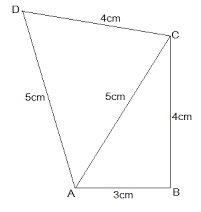In $$ΔABC$$, consider

$$AB^2 + BC^2 = 3^2 + 4^2 = 5^2 = AC^2$$

Since it obeys Pythagoras theorem we can say $$\Delta ABC$$ is right angled at $$B$$

Since $$\Delta ABC$$ is right angled at $$B$$,

Area of $$\Delta ABC$$

\begin{align}&={\frac{1}{2} \times \text { base } \times \text { height }} \\ {\qquad}&={\frac{1}{2} \times 3 \times 4}\end{align}

Area of $$\Delta ABC$$ $$= 6 \;\rm{cm^2}$$

In $$\Delta ADC,$$ we have,

$$\rm{a =} 5 \;\rm{cm,} \rm{b} = 4 \;\rm{cm }$$ and $$\rm{ c} = 5 \;\rm{cm}$$

Semi Perimeter

\begin{align}S&=\frac{(a+b+c)}{2} \\& =\frac{5+4+5}{2} \\ & =\frac{14}{2} \\ & =7 \mathrm{cm}\end{align}

By using Heron’s formula,

Area of  $$\Delta \, ADC$$

\begin{align} &={\sqrt{s(s-a)(s-b)(s-c)}} \\&=\sqrt{7(7-5)(7-4)(7-5)} \\ &=\sqrt{7 \times 2 \times 3 \times 2} \\ &=\sqrt{84} \mathrm{cm}^{2} \\ &=9.2 \mathrm{cm}^{2} \end{align}

Area of the quadrilateral $$ABCD$$

\begin{align} &= \text{Area of} \,\Delta ADC + \text{Area of} \, \Delta ABC \\ & = 9.2 \;\rm{cm}^2 + 6 \;\rm{cm}^2 \\ & = 15.2 \;\rm{cm}^2 \end{align}

## Chapter 12 Ex.12.2 Question 3

Radha made a picture of an aeroplane with coloured paper as shown in Figure. Find the total area of the paper used..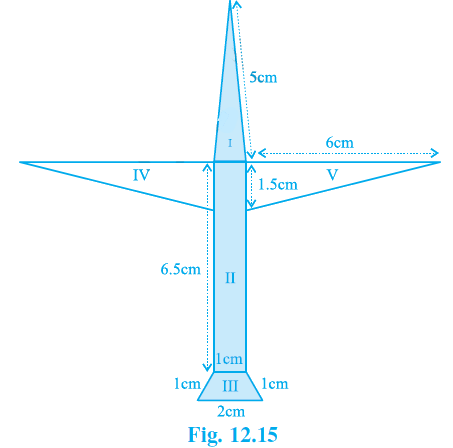### Solution

What is known?

Dimensions of the included figures in the given polynomial.

What is unknown?

Area of the triangle, rectangle, trapezium.

Reasoning:

We can calculate the area of polygon by dividing the polygon into triangular and quadrilateral parts and then use the Heron’s formula for area of the triangle and general formula for trapezium and rectangle.

The formula given by Heron about the area of a triangle

$$=\sqrt{s(s-a)(s-b)(s-c)}$$

Where $$a, b$$ and $$c$$ are the sides of the triangle, and

\begin{align}s &= \text{Semi-perimeter}\\& = \begin{Bmatrix} \text{Half the Perimeter } \\ \text{ of the triangle} \;\end{Bmatrix} \\&=\frac{(a+b+c)}{2}\end{align}

Steps:

(i) For the triangle marked I:

Sides $$a = 5 \;\rm{cm}$$, $$b = 5\; \rm{cm}$$, $$c = 1 \;\rm{cm}$$

Semi Perimeter

\begin{align}s & =\frac{(a+b+c)}{2} \\ & =\frac{5+5+1}{2} \\ & =\frac{11}{2}\\ & =5.5 \mathrm{cm}\end{align}

By using Heron’s formula,

Area of triangle I

\begin{align}&={\sqrt{s(s-a)(s-b)(s-c)}} \\&=\sqrt{ 5.5(5.5\!-\!5) (5.5\!-\!5) (5.5\!-\!1)} \\ &=\sqrt{5.5 \times 0.5 \times 0.5 \times 4.5} \\ &=\sqrt{6.1875}\, {cm}^{2} \\ &=2.5\, {cm}^{2} \end{align}

Area of a triangle $$= 2.5 \;\rm{cm}^2$$

(ii) For the rectangle marked II

Area of Rectangle $$=$$ Length $$\times$$ Breadth

\begin{align}&=6.5 \,\mathrm{cm} \times 1 \,\mathrm{cm} \\ &=6.5 \,\mathrm{cm}^{2} \end{align}

(iii) For the trapezium marked III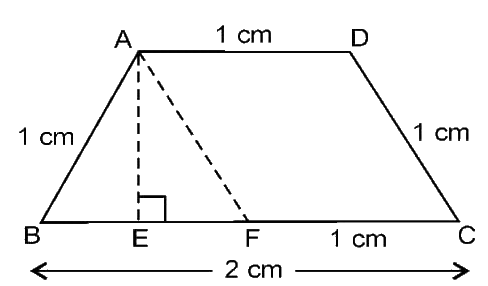Draw $$A E \perp B C$$ and $$AF$$ parallel to $$DC$$

\begin{align} & {AD}={FC}=1 \mathrm{cm}, \\ &AB= DC =1 \mathrm{cm},\\ &BC =2 \mathrm{cm},\\ & AF= DC =1 \mathrm{cm} .\end{align}

\begin{align} {BF}&={BC-FC} \\ &= 2 \mathrm{cm}-1 \mathrm{cm} \\ &=1 \mathrm{cm} \end{align}

Here $$\Delta ABF$$ is equilateral triangle, $$E$$ is the mid-point of $$BF$$

So, $$\rm{BE = EF}$$

\begin{align}\frac{\mathrm{BF}}{2}=\frac{1}{2}=0.5 \mathrm{cm}\end{align}

In $$\Delta AEF,$$

\begin{align} AF^{2} &=AE^{2}+EF^{2} \begin{bmatrix} \! \text { Pythagoras } \\ \!\!\text{theorem }\end{bmatrix} \\ 1^{2} &={AE}^{2+} 0.5^{2}\\{AE}^{2}&=\sqrt{1^{2}-0.5^{2}} \\ {AE}^{2} &=\sqrt{0.75} \\ {AE} &=0.9 {cm}\,\rm{}(approx) \end{align}

Area of trapezium

= $$\frac{1}{2} \, \times$$ sum of parallel sides  $$\times$$  distance between them

\begin{align} &=\frac{1}{2} \times({BC}+{AD}) \times {AE} \\ &=\frac{1}{2} \times 3 \times 0.9\end{align}

Area of trapezium $$= 1.4 \;\rm{cm}^2\rm{}(approx)$$

(iv) For the triangle marked IV and V

Triangles IV and V are congruent right angled triangles with base $$6 \;\rm{cm}$$ and height $$1.5 \rm{cm.}$$

Area of the triangles

\begin{align} &={\frac{1}{2} \times \text { base } \times \text { height }} \\ {\qquad}&{=\frac{1}{2} \times 6 \times 1.5} \\ &=4.5 \mathrm{cm}^{2}\end{align}

Area of two (IV & V) triangles$$= 4.5 \;\rm{cm}^2 + 4.5 \;\rm{cm}^2 = 9 \;\rm{cm}^2$$

Total area of paper used

\begin{align}&= \begin{Bmatrix} 2.5\, \mathrm{cm}^{2}\,+\,6.5 \,\mathrm{cm}^{2} \\ + 1.4 \mathrm{cm}^{2}\,+\,9 \,\mathrm{cm}^{2} \end{Bmatrix} \\ &=19.4 \,\mathrm{cm}^{2} \end{align}

## Chapter 12 Ex.12.2 Question 4

A triangle and a parallelogram have the same base and the same area. If the sides of the triangle are $$26\; \rm{cm}$$, $$28 \;\rm{cm}$$ and $$30 \;\rm{cm}$$, and the parallelogram stands on the base $$28 \;\rm{cm}$$, find the height of the parallelogram.

### Solution

What is known?

Dimensions of the triangle and base of parallelogram.

What is unknown?

Height of the parallelogram.

Reasoning:

By using Heron’s formula calculate the area of triangle and it is given that area of triangle is equal to area of parallelogram.

The formula given by Heron about the area of a triangle

$$=\sqrt{s(s-a)(s-b)(s-c)}$$

Where $$a, b$$ and $$c$$ are the sides of the triangle, and

\begin{align}s &= \text{Semi-perimeter}\\& = \begin{Bmatrix} \text{Half the Perimeter } \\ \text{ of the triangle} \;\end{Bmatrix} \\&=\frac{(a+b+c)}{2}\end{align}

Steps:

$$ABCD$$ is parallelogram.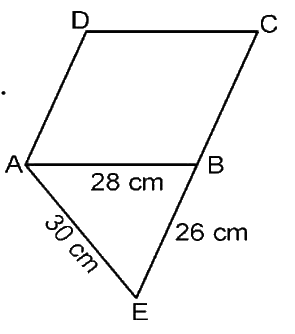$$ABE$$ is a triangle having base common with parallelogram $$ABCD.$$

For $$\Delta ABE,$$

$$a = 30\;{\rm{ cm}} , b = 26 \;{\rm{ cm}} , c = \;28 \;cm$$

Semi Perimeter

\begin{align}s&=\frac{(a+b+c)}{2} \\&=\frac{30+26+28}{2} \\ & =\frac{84}{2} \\ &=42 \mathrm{cm}\end{align}

By using Heron’s formula,

Area of a triangle $$ABE$$

\begin{align}\text{}&={\sqrt{s(s-a)(s-b)(s-c)}} \\ &=\sqrt{ 42(42-30) (42-28) (42-26) } \\ &=\sqrt{42 \times 12 \times 14 \times 16} \\ &=336 \mathrm{cm}^{2} \end{align}

Area of parallelogram $$ABCD$$ $$=$$ Area of  $$\Delta \, ABE$$,

\begin{align} \rm{Base} \times \rm{Height}&= 336 \;\rm{cm}^2\\ 28\; \rm{cm} \times Height& = 336 \;\rm{cm}^2\\ \text{Height}&=\frac{336 \mathrm{cm}^{2}}{28 \mathrm{cm}} \end{align}

Height of parallelogram $$= 12 \;\rm{cm}$$

## Chapter 12 Ex.12.2 Question 5

A rhombus shaped field has green grass for $$18$$ cows to graze. If each side of the rhombus is $$30 \;\rm{m}$$ and its longer diagonal is $$48 \;\rm{m}$$, how much area of grass field will each cow be getting?

### Solution

What is known?

Dimensions of the rhombus.

What is unknown?

Area of grass field that each Cow be getting.

Reasoning:

By using Heron’s formula calculate the area of triangle and then area of rhombus.

The formula given by Heron about the area of a triangle

$$=\sqrt{s(s-a)(s-b)(s-c)}$$

Where $$a, b$$ and $$c$$ are the sides of the triangle, and

\begin{align}s &= \text{Semi-perimeter}\\& = \begin{Bmatrix} \text{Half the Perimeter } \\ \text{ of the triangle} \;\end{Bmatrix} \\&=\frac{(a+b+c)}{2}\end{align}

Steps: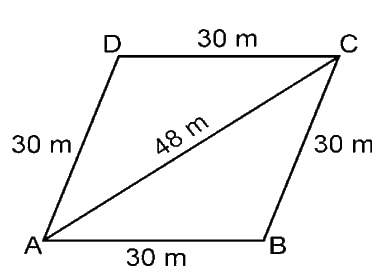The diagonal $$AC$$ divides the rhombus into two congruent triangles

For $$\Delta ABC$$

$$a = b =30 \;\rm{m}$$$$c = 48 \;\rm{m}$$

Semi Perimeter

\begin{align}s& =\frac{(a+b+c)}{2} \\ & =\frac{30+30+48}{2}\\ & =\frac{108}{2} \\ & =54 \, \mathrm{m}\end{align}

By using Heron’s formula,

Area of $$\Delta \, ABC$$

\begin{align} &={\sqrt{s(s-a)(s-b)(s-c)}} \\ &=\sqrt{54(54-30) (54-30) (54-48) } \\ &=\sqrt{54 \times 24 \times 24 \times 6} \\ &=432 \mathrm{m}^{2}\end{align}

Area of rhombus

\begin{align} &= \text{Area of a }\Delta \, ABC \\&=2 \times 432 \,\mathrm{m}^{2}\\&=864\, \mathrm{m}^{2} \end{align}

Number of cows $$=18$$

Area of grass field will each cow get $$= \frac{864 \mathrm{m}^{2}}{18}$$

Each cow gets an area of grass $$=48 \;\rm{m}^2.$$

## Chapter 12 Ex.12.2 Question 6

An umbrella is made by stitching $$10$$ triangular pieces of cloth of two different colors See Figure, each piece measuring $$20 \;\rm{cm}$$, $$50\;\rm{ cm}$$ and $$50\;\rm{ cm}$$. How much cloth of each color is required for the umbrella?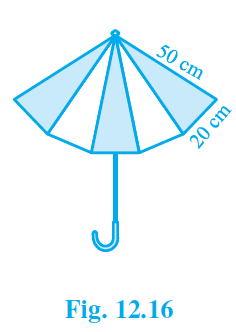### Solution

What is known?

Dimensions of the each triangular piece used in umbrella.

What is unknown?

Each color of cloth required for the umbrella.

Reasoning:

By using Heron’s formula we can calculate the area of triangle.

The formula given by Heron about the area of a triangle

$$=\sqrt{s(s-a)(s-b)(s-c)}$$

Where $$a, b$$ and $$c$$ are the sides of the triangle, and

\begin{align}s &= \text{Semi-perimeter}\\& = \begin{Bmatrix} \text{Half the Perimeter } \\ \text{ of the triangle} \;\end{Bmatrix} \\&=\frac{(a+b+c)}{2}\end{align}

Steps:

We know that umbrella is made of $$10$$ triangular pieces of cloth of two different colours.

Let us calculate the area of one triangle.

For $$\Delta ABC$$,

$$a = b = 50\; {\rm{cm}}, c = 20 \;{\rm{cm}}$$

Semi Perimeter

\begin{align}s&=\frac{(a+b+c)}{2} \\ & =\frac{50+50+20}{2} \\ & =\frac{120}{2} \\ & =60 \mathrm{cm}\end{align}

By using Heron’s formula,

Area of  $$\Delta \, ABC$$

\begin{align} &={\sqrt{s(s-a)(s-b)(s-c)}} \\ &=\sqrt{ 60(60-50) (60-50) (60-20) } \\ &=\sqrt{60 \times 10 \times 10 \times 40} \\ &=200 \sqrt{6} \mathrm{cm}^{2} \end{align}

Therefore area of $$10$$  triangular pieces

\begin{align}&{=10 \times 200 \sqrt{6} \mathrm{cm}^{2}} \\ &{=2000 \sqrt{6} \mathrm{cm}^{2}}\end{align}

Hence cloth required for each color

\begin{align}& =\frac{2000 \sqrt{6} \mathrm{cm}^{2}}{2} \\ & =1000 \sqrt{6} \mathrm{cm}^{2}\end{align}

## Chapter 12 Ex.12.2 Question 7

A kite in the shape of a square with a diagonal $$32 \;\rm{cm}$$ and an isosceles triangle of base $$8 \;\rm{cm}$$ and sides $$6\; \rm{cm}$$ each is to be made of three different shades as shown in Figure How much paper of each shade has been used in it?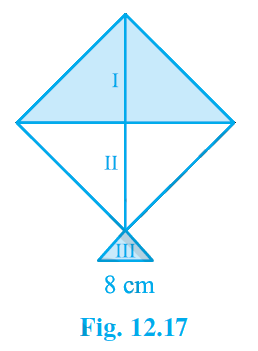### Solution

What is known?

Dimensions of the square used to prepare kite.

What is unknown?

Paper used in each shade to make kite.

Reasoning:

By using Heron’s formula calculate the area of each triangular shade.

The formula given by Heron about the area of a triangle

\begin{align}=\sqrt{s(s-a)(s-b)(s-c)}\end{align}

Where $$a, b$$ and $$c$$ are the sides of the triangle, and

\begin{align}s &= \text{Semi-perimeter}\\& = \begin{Bmatrix} \text{Half the Perimeter } \\ \text{ of the triangle} \;\end{Bmatrix} \\&=\frac{(a+b+c)}{2}\end{align}

Steps:

We know that diagonals of square bisect each other at right angle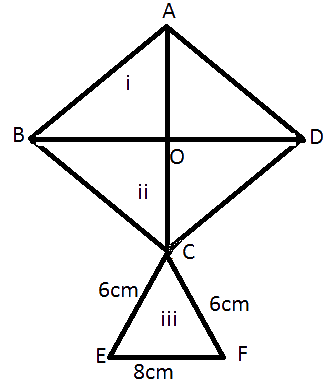Given diagonal $$\rm{BD = AC = 32 \;cm}$$,

then OA = \begin{align}\frac{1}{2}\end{align}, $$\rm{AC} = 16 \;\rm{cm}$$

Isosceles triangle of base $$32 \rm\,{cm}$$ and sides $$16 \rm\,{cm}$$

$$\Delta ABD$$ is right angled triangle.

Area of $$\Delta \, ABD$$

\begin{align} &={\frac{1}{2} \times \text { base } \times \text { height }} \\ &=\frac{1}{2} \times 32 \times 16 \\ &=256\, \mathrm{cm}^{2} \end{align}

Since the triangles that divides the square into two parts are equal.

Therefore

Area of $$\Delta ABD$$= Area of $$\Delta BCD$$ $$= 256 \;\rm{cm}^2$$

For $$\Delta CEF$$

Semi Perimeter

\begin{align}s &=\frac{(a+b+c)}{2}\\ & =\frac{6+6+8}{2} \\ & =\frac{20}{2} \\ & =10\, \mathrm{cm} \end{align}

By using Heron’s formula,

Area of $$\Delta \, CEF$$

\begin{align} &={\sqrt{s(s-a)(s-b)(s-c)}} \\ &=\sqrt{ 10(10-6) (10-6) (10-8) } \\ &=\sqrt{10 \times 4 \times 4 \times 2} \\ &=\sqrt{320} \\ & {=17.92 \,\mathrm{cm}^{2}}\end{align}

Paper used to make shade I $$= 256 \;\rm{cm}^2$$,

shade II $$= 256 \;\rm{cm}^2$$,

shade III $$= 17.92 \;\rm{cm}^2.$$

## Chapter 12 Ex.12.2 Question 8

A floral design on a floor is made up of $$16$$ tiles which are triangular, the sides of the triangle being $$9\; \rm{cm}$$, $$28\;\rm{ cm}$$ and $$3\; \rm{cm}$$ see Figure.Find the cost of polishing the tiles at the rate of $$50 \,\rm p$$ per $$\rm cm^2.$$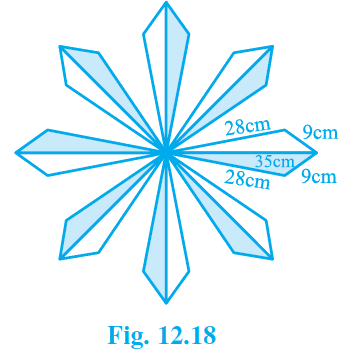### Solution

What is known?

Dimensions of the tiles(triangle).

What is unknown?

Total area of floral design and cost of polishing the tiles.

Reasoning:

By using Heron’s formula calculate the area of triangle.

The formula given by Heron about the area of a triangle

\begin{align}=\sqrt{s(s-a)(s-b)(s-c)}\end{align}

Where $$a, b$$ and $$c$$ are the sides of the triangle, and

\begin{align}s &= \text{Semi-perimeter}\\& = \begin{Bmatrix} \text{Half the Perimeter } \\ \text{ of the triangle} \;\end{Bmatrix} \\&=\frac{(a+b+c)}{2}\end{align}

Steps:

We have the dimensions of sides of one triangular tile.

$$a = 35\; {\rm{cm}}, b = 28 \;{\rm{cm}}, c = 9 \;{\rm{cm}}$$

Semi Perimeter

\begin{align}s&=\frac{(a+b+c)}{2}\\ & =\frac{35+28+9}{2} \\ & =\frac{72}{2} \\ & =36 \mathrm{cm}\end{align}

By using Heron’s formula,

Area of  triangular tile

\begin{align}\text{}&={\sqrt{s(s-a)(s-b)(s-c)}} \\ &=\sqrt{ 36(36-35) (36-28) (36-9) } \\ &=\sqrt{36 \times 1 \times 8 \times 27} \\ &=36 \sqrt{6} \,\mathrm{cm}^{2}\end{align}

Area of a $$1$$ triangular tile $$=36 \sqrt{6} \,\mathrm{cm}^{2}$$

Area of $$16$$ triangular tile

\begin{align} &=16 \times 36 \sqrt{6} \,\mathrm{cm}^{2} \\ & = 1410.90 \,\mathrm{cm}^{2} \end{align}

Cost of polishing $$1\;\rm cm^2$$ of tile is $$50 \, \rm p$$

Therefore cost of $$1410.90 \;\rm cm^2$$ of tile is

$$= 1410.90 \;\rm cm^2 \times \; 0.5$$

$$={\rm{Rs}} \, 705.45 \,\,{ \rm{approx}}$$

## Chapter 12 Ex.12.2 Question 9

A field is in the shape of a trapezium whose parallel sides are $$25 \;\rm m$$ and $$10 \;\rm m$$. The non-parallel sides are$$14\;\rm m$$ and $$13 \;\rm m$$. Find the area of the field.

### Solution

What is known?

Dimensions of the field (trapezium).

What is unknown?

Area of field (trapezium).

Reasoning:

Using Heron’s formula find the area of triangle and then using that area, we can find the height of the trapezium and hence its area.

The formula given by Heron about the area of a triangle

$$=\sqrt{s(s-a)(s-b)(s-c)}$$

Where $$a, b$$ and $$c$$ are the sides of the triangle, and

\begin{align}s &= \text{Semi-perimeter}\\& = \begin{Bmatrix} \text{Half the Perimeter } \\ \text{ of the triangle} \;\end{Bmatrix} \\&=\frac{(a+b+c)}{2}\end{align}

Steps:

Given $$ABCD$$ is field. Draw \begin{align}C G \perp A B\end{align} and $$CF$$ parallel to $$DA$$.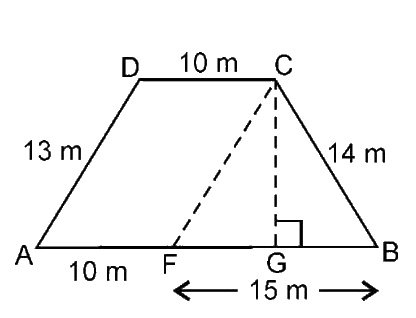$$DC = AF = 10 \; \rm m,$$

$$DA = CF = 13 \; \rm m$$

$${\rm{So}}, FB = 15 \; \rm m$$

In $$\Delta CFB$$

$$a = 15\; \rm m$$$$b = 14\; \rm m$$$$c = 13 \; \rm m.$$

Semi Perimeter

\begin{align}s & =\frac{(a+b+c)}{2}\\&=\frac{15+14+13}{2}\\ &=\frac{42}{2} \\ &=21 \mathrm{m}\end{align}

By using Heron’s formula,

Area of $$\Delta \, CFB$$

\begin{align} &={\sqrt{s(s-a)(s-b)(s-c)}} \\ &=\sqrt{ 21(21-15) (21-14) (21-13) } \\ &=\sqrt{21 \times 6 \times 7 \times 8} \\ &=84 \, \mathrm{m}^{2}\end{align}

Also the area of $$\Delta \,CFB$$

\begin{align}=\frac{1}{2} \times \text { base } \times \text{height}\end{align}

\begin{align}84&=\frac{1}{2} \times {BF} \times {CG} \\ 84&=\frac{1}{2} \times 15 \times {CG} \\ {{CG}}&={\frac{84 \times 2}{150}=11.2 {m}}\end{align}

Area of trapezium

$$=\frac{1}{2} \times$$ sum of parallel sides $$\times$$ distance between them

\begin{align} &=\frac{1}{2} \times(25+10) \times 11.2 \\ &=196\, \mathrm{m}^{2} \end{align}

Hence the area of field is $$196 \rm \;m^2.$$

Instant doubt clearing with Cuemath Advanced Math Program